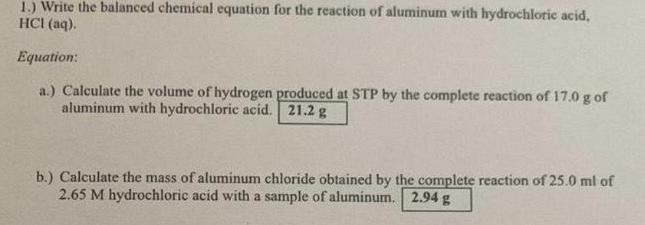Question:

# Write the balanced chemical equation for the reaction of

Last updated: 8/6/2022Write the balanced chemical equation for the reaction of aluminum with hydrochloric acid, HCI (aq). Equation: a.) Calculate the volume of hydrogen produced at STP by the complete reaction of 17.0 g of aluminum with hydrochloric acid. b.) Calculate the mass of aluminum chloride obtained by the complete reaction of 25.0 ml of 2.65 M hydrochloric acid with a sample of aluminum.